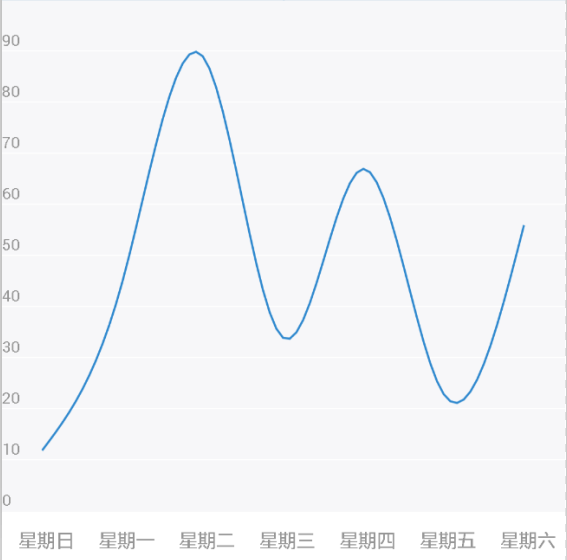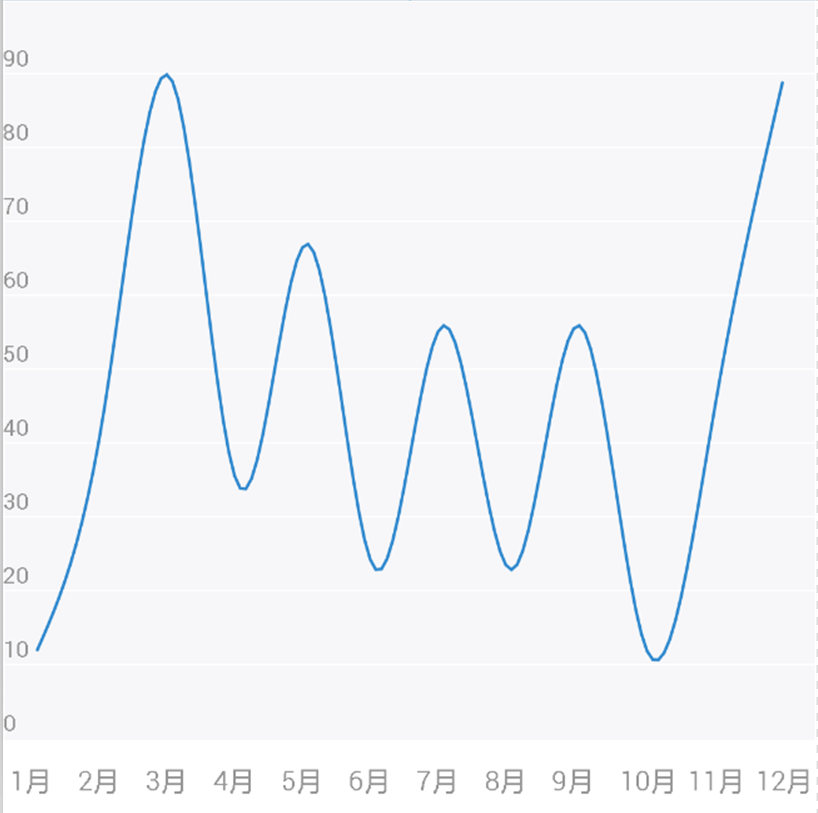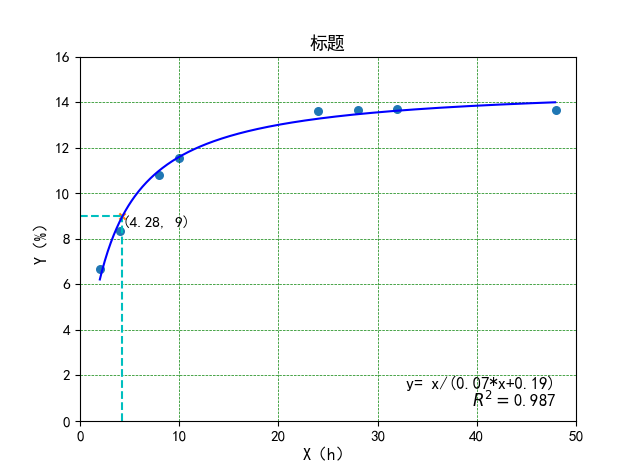• 2021-01-14 01:24:04不知你要画什么样的坐标图,下面是怎么样在WORD文档中画坐标曲线图

1.执行“绘图/绘图网格/在屏幕上显示网格(注意数据设置)/确定”命令，使页面上显示绘图网格；

2.用“绘图”工具栏上的“箭头”画坐标轴，画时按住Shift键，保证画出的线水平、垂直；

3.用“直线”或“椭圆”画坐标轴上的刻度；

4.用“本框”制作数轴上数据标识；

5.把直线、刻度、数据标识同时选中并右击，执行“组合/组合”命令，把所有图形组合起来成为一个完整的平面直角坐标系，便于整体移动摆放。

6.用“椭圆”制作坐标点(画时按住Shift键)、用“直线”画点向坐标轴引的垂线、用“文本框”制作点的坐标。

7.用“绘图”工具栏上的“自选图形”制作函数图形。

1、绘图工具栏打开后，选“箭头”，用它横着拖出一条带箭头(它的形状、大小均可以在“设置图形格式”里面更改)的直线，要想拖得很直，拖动同时按住shift键。 2、用同样的方法拖出一条带箭头的竖线。 3、将两条线同时选中，点击绘图工具栏最左边的“绘图”按钮，分别使用“对齐与分布”中的“左对齐”、“底端对齐”将它俩的位置调整好。如果觉得大小合适，就在此时将它们“组合”起来。 4、用绘图中的“直线”拖出一条短竖线，觉得长短、粗细、颜色合适就复制若干个，再将它们全部选中，用刚才的方法将它们底端对齐而且“横向分布”。此时，横坐标上的刻度就初具雏形了，将它们组合，再选中刚才已经组合的坐标再将它们底端对齐，调整位置。 5、在绘图中选“艺术字”，做一个大小、内容适合你的数字或字母或文字的标注，复制若干个，将它们编辑好序列，放大显示比例，将第一个标注尽量拖到你的第一个刻度(或0刻度)下边(要想精确移动，按住Ctrl键再按上、下、左、右键)合适的位置，将最后一个标注拖到最后一个刻度下边，然后将所有的标注(不论其位置如何)选中，使它们底端对齐且横向分布，再移到合适的位置。横向坐标连标注都OK啦，纵向的照此办理即可。最后如果你觉得满意了，将所有的内容全选中了，组合！整个坐标轴的内容将可以任意拖动和调整大小，插入在你需要的地方。

◆◆

评论读取中....

请登录后再发表评论!

◆◆

修改失败，请稍后尝试

更多相关内容
• 在winform界面上画坐标，360的箭头，曲线等 在winform界面上画坐标，360的箭头，曲线等 在winform界面上画坐标，360的箭头，曲线等 在winform界面上画坐标，360的箭头，曲线
• 实现QtCharts曲线图移动和缩放。这个资源描述怎么就要求要50个字了嘿
• 图片取点获取对应坐标参数工具，适用于根据给定坐标图曲线来获取精确曲线上点的x、y坐标，适用于学校或科研机构逆向从曲线上扒参数
• android自定义坐标曲线图

编程一年出头了，总是从别人的博客上学习东西，也想把自己的收获分享出去。

第一次写博客，废话不多说，android 自定义坐标曲线图

先上效果图：

第一张(每周数据)：第二张(每月数据)：两张图其实就是数据容量不一样，实现的道理是完全相同的。

先声明，曲线的实现参考了别人的方法，并非原创，此部分详细请见android画经过多点的曲线

好了，简单分析一下图的构成：

1、横坐标文字。2、纵坐标数字。3、上面大部分灰色背景和下部白色背景。4、与x轴平行的白色标度细线。5、最核心的部分，图中的蓝色曲线。

简单理一下实现步骤：

继承View类，然后：1.测控件的尺寸，分好比例。2.在计算好的位置画第一到第四部分。3.参考画曲线的方法，根据数据设置画蓝色曲线。

第一步，在重写onWindowFocusChanged方法中获取控件的宽高尺寸，根据当前的模式，将宽和高分为相应的份数。这里高分为11份，宽分为7份或12份(对应周数据模式和月数据模式)。这里注意不要在构造方法中用getWidth()和getHeight()方法获取宽高尺寸，此时控件还未完成绘制，你获得的所有尺寸都会是0。在activity的onCreate()方法中做此操作也是一样道理，获取的尺寸都是0。

第二步，在ondraw方法中在计算好的位置，在Canvas上用相应的Paint画文字、数字、背景、标度线。注意paint设置不同的颜色，粗细，填充模式。

第三步，依旧在ondraw方法中，根据数据和显示模式画曲线。

然后留出最基本的设置接口：1是设置显示模式，2是设置y轴上的数据集合，就基本完成了。

这里还有一点需要引起极大注意的是：设置数据时一定要保证控件已经获得焦点并且正确的计算出了相关尺寸，否则在没有正确基准尺寸的情况下计算显示出来的图像必定是异常的。这也是为什么代码中设置y轴数据时如果没有焦点要delay 150ms再执行相关操作的原因。如果在activity oncreate时就设置数据并且计算显示，获取的基本尺寸为0，那么肯定什么也显示不出来的。

x轴暂时只有两个尺度模式，y轴的尺度也默认是0~100,这个具体需求具体改变，比如实际项目中就有传过来1000以上的数字，且x轴要显示几月几日，这时候，数字尺度和单位都要做修改，甚至要扩展新的显示模式了，不过最基本的显示架子就是这样，具体有什么需求可以根据实际情况做改变了。

下面贴出代码

package com.epuxun.ewater.view;

import java.util.ArrayList;
import java.util.List;

import android.R.integer;
import android.content.Context;
import android.graphics.Canvas;
import android.graphics.Color;
import android.graphics.Paint;
import android.graphics.Paint.Style;
import android.graphics.Path;
import android.os.Handler;
import android.util.AttributeSet;
import android.util.Log;
import android.view.View;

/**
* 曲线坐标图
* @author zhangyu
* @date 2016-3-8
*/
public class CurveChartView extends View {

private static final String TAG = "CurveChartView";
private final int STEP = 12;
private boolean haveFocuse = false;
// 曲线图显示模式
private int model;
// 按周显示
public static final int MODEL_WEEK = 0;
// 控件宽高
private int viewWidth, viewHeigth;
// 每一份的水平、竖直尺寸
private float averageWidth, averageHeigth;
// 画笔
Paint grayPaint, bluePaint, blackPaint, whitePaint;
private String[] weekDay = new String[] { "日", "一", "二", "三", "四", "五", "六" };
// x、y轴坐标值
private List<Integer> pointX, pointY;
// 曲线路径
private Path curvePath;

public CurveChartView(Context context) {
super(context);
init();
}

public CurveChartView(Context context, AttributeSet attrs) {
super(context, attrs);
init();
}

public CurveChartView(Context context, AttributeSet attrs, int defStyleAttr) {
super(context, attrs, defStyleAttr);
init();
}

private void init() {
model = MODEL_WEEK;// 默认为显示周数据
grayPaint = new Paint();
grayPaint.setColor(Color.parseColor("#F7F7F9"));
grayPaint.setStrokeWidth(1.5f);
bluePaint = new Paint();
bluePaint.setColor(Color.parseColor("#2E88CE"));
bluePaint.setAntiAlias(true);
bluePaint.setStyle(Paint.Style.STROKE);
blackPaint = new Paint();
blackPaint.setColor(Color.parseColor("#969696"));
blackPaint.setStrokeWidth(3.0f);
whitePaint = new Paint();
whitePaint.setColor(Color.parseColor("#ffffff"));
whitePaint.setStrokeWidth(2.5f);

pointX = new ArrayList<Integer>();

curvePath = new Path();

for (int i = 0; i < pointX.size(); i++) {
Log.i(TAG, "init..  pointX[" + i + "] = " + pointX.get(i) + ",pointY[" + i + "] = " + pointY.get(i));
}
Log.d(TAG, "init..");
}

/**
* 设置显示模式 0为显示一周每天数据 否则为显示每月数据
* @param model
*/
public void setShowModel(int model) {
this.model = model;
initMeasure();
pointX.clear();
if (model == MODEL_WEEK)
for (int i = 0; i < 7; i++) {
pointX.add((int) ((i + 0.5) * averageWidth));
}
else
for (int i = 0; i < 12; i++)
pointX.add((int) ((i + 0.5) * averageWidth));
invalidate();
}

/**
* 设置y坐标上的值
* @param pointY
*/
public void setPointsY(final List<Integer> pointY1) {
if (null != pointY1) {
if(!haveFocuse)
new Handler().postDelayed(new Runnable() {
@Override
public void run() {
setYDatas(pointY1);
}
}, 180);
else
setYDatas(pointY1);
}
}

private void setYDatas(final List<Integer> pointY1) {
pointY = pointY1;
for(int i = 0;i < pointY.size();i++)
pointY.set(i, transformYCoordinate(pointY.get(i)));
invalidate();
}

/**
* 将传入的y数据值转换为屏幕坐标值
*
* @param y
* @return
*/
public int transformYCoordinate(int y) {
Log.v(TAG, "transformYCoordinate..  viewHeigth = " + viewHeigth);
return (int) (viewHeigth - ((y / 100f) * viewHeigth));
}

@Override
protected void onDraw(Canvas canvas) {

drawGrayBackground(canvas);
drawYCoordinateNumber(canvas);
drawYCoordinateLine(canvas);
drawXCoordinateText(canvas);
drawCurveChart(canvas);

super.onDraw(canvas);
}

/**
* 画灰、白色背景
*/
private void drawGrayBackground(Canvas canvas) {
Log.d(TAG, "drawGrayBackground..viewWidth = " + viewWidth + ",viewHeigth = " + viewHeigth);
grayPaint.setStyle(Style.FILL);
whitePaint.setStyle(Style.FILL);

canvas.drawRect(0, 0, viewWidth, viewHeigth * (10f / 11), grayPaint); // 灰色背景
canvas.drawRect(0, viewHeigth * (10f / 11), viewWidth, viewHeigth, whitePaint); // 白色背景

}

/**
* 画曲线
*
* @param canvas
*/
private void drawCurveChart(Canvas canvas) {
curvePath.reset();

List<Cubic> calculate_x = calculate(pointX);
List<Cubic> calculate_y = calculate(pointY);

if (null != calculate_x && null != calculate_y && calculate_y.size() >= calculate_x.size()) {
curvePath.moveTo(calculate_x.get(0).eval(0), calculate_y.get(0).eval(0));

for (int i = 0; i < calculate_x.size(); i++) {
for (int j = 1; j <= STEP; j++) {
float u = j / (float) STEP;
curvePath.lineTo(calculate_x.get(i).eval(u), calculate_y.get(i).eval(u));
}
}
}

bluePaint.setStrokeWidth(4);
bluePaint.setStrokeWidth(4);
canvas.drawPath(curvePath, bluePaint);

}

/**
* 画Y坐标上横线
*
* @param canvas
*/
private void drawYCoordinateLine(Canvas canvas) {
for (int i = 1; i < 10; i++)
canvas.drawLine(0, averageHeigth * i, viewWidth, averageHeigth * i, whitePaint);
}

/**
* 画x坐标文字
* @param canvas
*/
private void drawXCoordinateText(Canvas canvas) {
blackPaint.setTextSize(DisplayUtils.sp2px(getContext(), 12));
if (model == MODEL_WEEK)
for (int i = 0; i < 7; i++)
canvas.drawText("星期" + weekDay[i], (0.2f + i) * averageWidth, 10.7f * averageHeigth, blackPaint);
else {
for (int i = 0; i < 12; i++)
canvas.drawText(i + 1 + "月", (0.1f + i) * averageWidth, 10.7f * averageHeigth, blackPaint);
}
}

/**
* 画y轴坐标值
* @param canvas
*/
private void drawYCoordinateNumber(Canvas canvas) {
Log.d(TAG, "drawYCoordinateNumber..");
blackPaint.setTextSize(DisplayUtils.sp2px(getContext(), 10));
for (int i = 0; i < 10; i++)
canvas.drawText("" + (i * 10), viewWidth * (13 / 140), (float) (9.9 - i) * averageHeigth, blackPaint);
}

/**
* 计算曲线.
* @param x
* @return
*/
private List<Cubic> calculate(List<Integer> x) {
if (null != x && x.size() > 0) {
int n = x.size() - 1;
float[] gamma = new float[n + 1];
float[] delta = new float[n + 1];
float[] D = new float[n + 1];
int i;
/*
* We solve the equation [2 1 ] [D] [3(x - x) ] |1 4 1 |
* |D| |3(x - x) | | 1 4 1 | | . | = | . | | ..... | | . |
* | . | | 1 4 1| | . | |3(x[n] - x[n-2])| [ 1 2] [D[n]] [3(x[n] -
* x[n-1])]
*
* by using row operations to convert the matrix to upper triangular
* and then back sustitution. The D[i] are the derivatives at the
* knots.
*/

gamma = 1.0f / 2.0f;
for (i = 1; i < n; i++) {
gamma[i] = 1 / (4 - gamma[i - 1]);
}
gamma[n] = 1 / (2 - gamma[n - 1]);

delta = 3 * (x.get(1) - x.get(0)) * gamma;
for (i = 1; i < n; i++) {
delta[i] = (3 * (x.get(i + 1) - x.get(i - 1)) - delta[i - 1]) * gamma[i];
}
delta[n] = (3 * (x.get(n) - x.get(n - 1)) - delta[n - 1]) * gamma[n];

D[n] = delta[n];
for (i = n - 1; i >= 0; i--) {
D[i] = delta[i] - gamma[i] * D[i + 1];
}

/* now compute the coefficients of the cubics */
for (i = 0; i < n; i++) {
Cubic c = new Cubic(x.get(i), D[i], 3 * (x.get(i + 1) - x.get(i)) - 2 * D[i] - D[i + 1], 2 * (x.get(i) - x.get(i + 1)) + D[i] + D[i + 1]);
}
return cubics;
}
return null;
}

public class Cubic {

float a, b, c, d; /* a + b*u + c*u^2 +d*u^3 */

public Cubic(float a, float b, float c, float d) {
this.a = a;
this.b = b;
this.c = c;
this.d = d;
}

/** evaluate cubic */
public float eval(float u) {
return (((d * u) + c) * u + b) * u + a;
}
}

@Override
public void onWindowFocusChanged(boolean hasWindowFocus) {
if (hasWindowFocus) {
haveFocuse = true;
initMeasure();
setShowModel(this.model);
}
super.onWindowFocusChanged(hasWindowFocus);
}

private void initMeasure() {
viewWidth = getWidth();
viewHeigth = getHeight();

averageHeigth = viewHeigth / 11;
if (model == MODEL_WEEK)
averageWidth = viewWidth / 7;
else
averageWidth = viewWidth / 12;

}
}


代码比较基础，结合注释应该很容易看明白，其中肯定还有多多少少的问题，欢迎大家指正，当然，也更希望对需要学习的同学有所帮助！

展开全文android 控件 自定义控件
• 演示Labview鼠标移动的同时在多个波形中绘制游标，并在右侧实时显示各个波形曲线X轴、Y轴坐标。应用场景：需要绘制的多曲线幅值差距太大，不得不分别绘制到多个波形中，此时又需要鼠标在波形图标中移动时又...
• C#基于DevExpress的chartcontrol做的画趋势曲线图：不断添加数据点，以曲线的方式显示在窗体上。DevExpress的chartcontrol默认是横坐标不断缩放，显示不太符合直观。改成了固定的横坐标，然后从左往右逐个添加数据点...
• python根据坐标点拟合曲线绘图 import os import numpy as np from scipy import log from scipy.optimize import curve_fit import matplotlib.pyplot as plt import math from sklearn.metrics import r2_...

# python根据坐标点拟合曲线绘图

任何程序错误，以及技术疑问或需要解答的，请添加import os
import numpy as np
from scipy import log
from scipy.optimize import curve_fit
import matplotlib.pyplot as plt
import math
from sklearn.metrics import r2_score
# 字体
plt.rcParams['font.sans-serif']=['SimHei']

# 拟合函数
def func(x, a, b):
#    y = a * log(x) + b
y = x/(a*x+b)
return y

# 拟合的坐标点
x0 = [2, 4, 8, 10, 24, 28, 32, 48]
y0 = [6.66,8.35,10.81,11.55,13.63,13.68,13.69,13.67]

# 拟合，可选择不同的method
result = curve_fit(func, x0, y0,method='trf')
a, b = result

# 绘制拟合曲线用
x1 = np.arange(2, 48, 0.1)
#y1 = a * log(x1) + b
y1 = x1/(a*x1+b)

x0 = np.array(x0)
y0 = np.array(y0)
# 计算r2
y2 = x0/(a*x0+b)
#y2 = a * log(x0) + b
r2 = r2_score(y0, y2)

#plt.figure(figsize=(7.5, 5))
# 坐标字体大小
plt.tick_params(labelsize=11)
# 原数据散点
plt.scatter(x0,y0,s=30,marker='o')

# 横纵坐标起止
plt.xlim((0, 50))
plt.ylim((0, round(max(y0))+2))

# 拟合曲线
plt.plot(x1, y1, "blue")
plt.title("标题",fontsize=13)
plt.xlabel('X（h）',fontsize=12)
plt.ylabel('Y（%）',fontsize=12)

# 指定点，y=9时求x
p = round(9*b/(1-9*a),2)
#p = b/(math.log(9/a))
p =  round(p, 2)
# 显示坐标点
plt.scatter(p,9,s=20,marker='x')
# 显示坐标点横线、竖线
plt.vlines(p, 0, 9, colors = "c", linestyles = "dashed")
plt.hlines(9, 0, p, colors = "c", linestyles = "dashed")
# 显示坐标点坐标值
plt.text(p, 9, (float('%.2f'% p),9),ha='left', va='top', fontsize=11)
# 显示公式
m = round(max(y0)/10,1)
print(m)
plt.text(48, m, 'y= x/('+str(round(a,2))+'*x+'+str(round(b,2))+')', ha='right',fontsize=12)
plt.text(48, m, r'$R^2=$'+str(round(r2,3)), ha='right', va='top',fontsize=12)

# True 显示网格
# linestyle 设置线显示的类型(一共四种)
# color 设置网格的颜色
# linewidth 设置网格的宽度
plt.grid(True, linestyle = "--", color = "g", linewidth = "0.5")
plt.show()
展开全文python 机器学习 开发语言
• 读取txt文件中的数字并在坐标图上绘制曲线，可以实现曲线不停绘制的效果
• ## C# 绘制实时曲线包括绘制坐标轴

千次下载 热门讨论 2014-08-06 02:08:35
C# 绘制实时曲线坐标轴，实现实时的图像处理操作。
• ## C#画坐标系及曲线

热门讨论 2012-09-05 16:29:05
程序中含一个Form，程序运行后，单击form的右下角就会出现图形 程序的可移植性较强
• Word中绘制曲线图的方法如下：1、通过桌面快捷方式或搜索来打开Word文档。2、在随后打开的界面左侧点击你想绘制曲线图的文档。3、在界面上方点击“插入”按钮，在随后打开的界面中点击“图表”按钮。4、在图标对话框...

Word中绘制曲线图的方法如下：

1、通过桌面快捷方式或搜索来打开Word文档。2、在随后打开的界面左侧点击你想绘制曲线图的文档。3、在界面上方点击“插入”按钮，在随后打开的界面中点击“图表”按钮。4、在图标对话框中选择“散点图”，之后选择“带平滑线的散点图”，之后点击“插入”。5、在自动打开的界面中输入自己的数据即可完成绘制。在word中如何画曲线？

1、首先在电脑上打开Word软件，接下来在Word中创建一个空白的文档。2、接下来在页面上方的工具栏中选择“插入”-“形状”，在选项中选择“曲线”。3、接下来在文档中用鼠标左键绘制自己需要的曲线即可。怎么样在WORD文档中画坐标曲线图

1.执行“绘图/绘图网格/在屏幕上显示网格(注意数据设置)/确定”命令，使页面上显示绘图网格；

2.用“绘图”工具栏上的“箭头”画坐标轴，画时按住Shift键，保证画出的线水平、垂直；

3.用“直线”或“椭圆”画坐标轴上的刻度；

4.用“本框”制作数轴上数据标识；

5.把直线、刻度、数据标识同时选中并右击，执行“组合/组合”命令，把所有图形组合起来成为一个完整的平面直角坐标系，便于整体移动摆放。

6.用“椭圆”制作坐标点(画时按住Shift键)、用“直线”画点向坐标轴引的垂线、用“文本框”制作点的坐标。

7.用“绘图”工具栏上的“自选图形”制作函数图形。

在Word中如何制做曲线图?

插入——对象——microsoft图表,然后选择自己所需类型

用WORD画图怎么画曲线

word 菜单 视图 工具栏 确保绘图是选中的 然后word窗口的下面出现绘图的工具栏，自选图形-线条，里面选你要的线条吧。

怎么做word曲线图

在视图工具栏里把自选图形勾出来，然后左击自选图形右下角的下拉三角形符号选择线条往右移再选择曲线，就可以在页面上自由的勾画出自己想画的曲线

如何在word中画曲线图？

先随便画一根直线状，然后点住线条击右键有个编辑顶点功能，然后按要求随便拉，如要圆弧形的，就击曲线段，就OK了

如何在WORD里面画曲线图

打开“插入”-“图片”-“图表”，然后编辑数据就可以得到你要的曲线图

展开全文• C# 坐标曲线图 观看横众坐标 C# 坐标曲线图 观看横众坐标
• python根据x轴、y轴坐标坐标轴里画出曲线图 程序实现： import numpy as np import matplotlib as mpl import matplotlib.pyplot as plt a=[1,2,3,5,6,10] b=[0,3,4,5,7,8] c=[10,9,8,7,6,5,2,0] d=[1,2,3,5,5,6...python 机器学习 开发语言
• 求Excel图表曲线上任意点的坐标.rar,在许多场合中经常有这样的问题：已知一组数据，并据此绘制了曲线图，如何求曲线上任意一点的坐标。如本例所示，如何根据已知的Y值，求X值。Excel应用
•matlab
• 工作需要实现一个双对数曲线图，没找到现成的插件，最后决定还是自己自绘一个，封装成插件算了。。。 最后的效果如下： 控件的属性设置如下为双对数曲线： 其他属性看说明即可，都在杂项里面比较简单。 ps:注意...chart
• 随机生成一组数据，QT用Qpainter绘制实时曲线图最简单的折线图
• python实现可暂停的动态曲线绘制，横纵坐标固定，同时保存数据到文件中
• ## 1.6 极坐标曲线

千次阅读 2020-05-21 19:18:20
坐标曲线作图的基本格式： polarplot(r(t), t=a…b) 例1.6.1 作出以下极坐标方程表示的曲线： 这是一个圆心在极点，半径为R的圆。 解 输入以下命令： with(plots): R:=1.5: polarplot(R, t=0…2*Pi, thickness=3)...
• C#WPF 如何绘制几何图形 图示教程 绘制sin曲线 正弦 绘制2D坐标系 有有代码 . 详细说明 http://blog.csdn.net/wyx100/article/details/42340217
• 一个能够绘制曲线图的控件,代码不完善,欢迎批评指正
• 三纵坐标图或者离心泵特性曲线的做法，origin三维制图方法~
• 0.引言在读文献的时，经常遇到这样的情况：文章里提出的方法好有趣啊，好想拿文中用的数据来试试看看能不能得到相近的结果，可是文中只有根据原始数据绘制的曲线图，没有数据。如下图所示。此时，如果能从文中把这幅...
• 有时候需要用到一些数据库里面曲线图的数据，进行进一步的变换处理，但是很多时候都只有图片，没有数据。基于这个问题，给出了以下算法。 思路：  1）通过图像算法中常用的边界识别的方法来识别曲线；  2）根据...计算机视觉 opencv 图像处理
• c# 坐标系控件，数据源dataTable,自动缩放
• 这是一个用VC++编写的画坐标曲线的程序，用户可以根据自己的需求更改其中的参数获得自己想要的曲线即可
•   在读文献的时，经常遇到这样的情况：文章里提出的方法好有趣啊，好想拿文中用的数据来试试看看能不能得到相近的结果，可是文中只有根据原始数据绘制的曲线图，没有数据。如下图所示。   此时，如果能从文中把...matlab
• ## 用UiBot做Excel曲线图

千次阅读 2020-12-21 14:44:06
曲线图坐标单位为每个数据间隔5分钟曲线图坐标以10为间隔。曲线图数据所在位置为b，c，d三行。项目过程：打开ub，新建流程，进入流程编辑。首先绑定需要打开的Excel文件，在数据后方插入横坐标间......